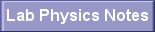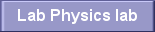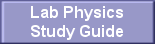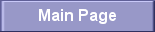LAB PHYSICS ASSIGNMENTS

ASSIGNMENTS

Introduction: A Physics Toolkit

SI Conversion Worksheet
Scientific Notation Worksheet
Significant Figures Worksheet
Problem Set 1A

Representing Motion in One Dimension

Finding the Average Velocity - 2A

Accelerated Motion

Average Acceleration - 2B
Displacement with Uniform Acceleration - 2C
Unit II: Worksheet 2
Unit II: Worksheet 3
Unit III: Worksheet 2
Free Fall Problem Worksheet

Newtonian Mechanics

Net External Force - 4A
Newton's Second Law - 4B

Work and Energy

Work - 5A
Kinetic Energy - 5B
Work - Kinetic Energy Theorem - 5C
Potential Energy - 5D
Conservation of Mechanical Energy - 5E
Power - 5F

Momentum

Worksheet 6A - Momentum
Worksheet 6B - Force and Momentum
Worksheet 6C - Stopping Distance
Worksheet  6D - Conservation of Momentum
Worksheet 6E - Perfectly Inelastic Collisions
Worksheet 6F - Kinetic Energy in Perfectly Inelastic Collisions
Worksheet 6G - Elastic Collisions

Rotational Motion and the Laws of Gravity

WS7I: Gravitation
WS7J: Kepler's Law
WS7E: Tangential Speed
WS7F: Tangential Acceleration
WS7G: Centripetal Acceleration
WS7H: Centripetal Force

Simple Harmonic Motion

WSA: Hooke's Law WSB: Simple Harmonic Motion of a Simple Pendulum
WSC: Simple Harmonic Motion of a Spring and Mass System
WSD: Wave Speed

Chapter 20: Mechanical Waves

WS14A: Intensity of Sound Waves
WS14B: Harmonics

Electric Charge, Force and Energy

WS17A: Electric Force - Coulomb's Law - Two Charges
WS17B: Electric Force - Coulomb's Law - More than two charges
WS17C: Equilibrium
WS17D: Electric Field
WS18A: Electric Potential Energy
WS18B: Potential Difference
WS18C: Capacitance

DC Circuits

WS19A: Current
WS19B: Resistance
WS19C: Electric Power
WS19D: Cost of Electrical Energy
WS20A: Resistors in Series
WS20B: Resistors in Parallel
WS20C: Resistors in Series and Parallel
WS20D: Current and Potential Difference across a Resistor

Light, Reflection, Mirrors, Refraction, Lenses, Interference,
Diffraction

Electromagnetic Waves 14A
Concave Mirrors 14B
Convex Mirrors 14C
Snell's Law 15A
Lenses 15B
Critical Angle
Interference
Diffraction GratingsDr. Richard T MalikMarlboro High School, N.J.rmalik@frhsd.com732-617-8393, ext. 6149
 LabPhysics